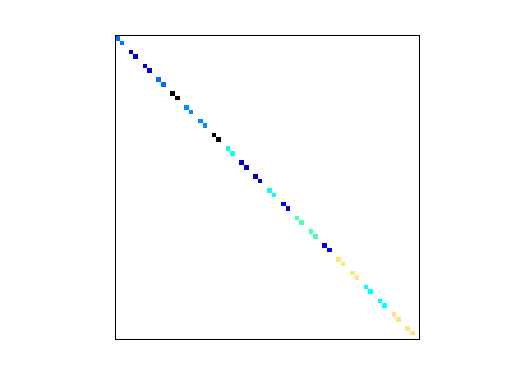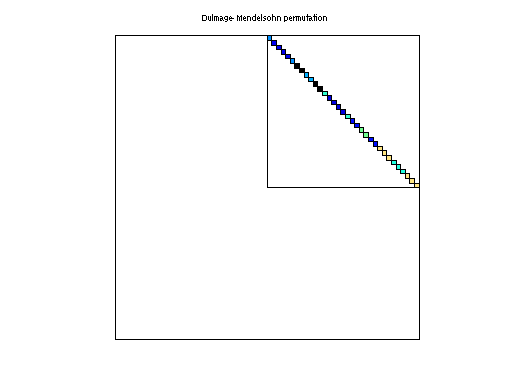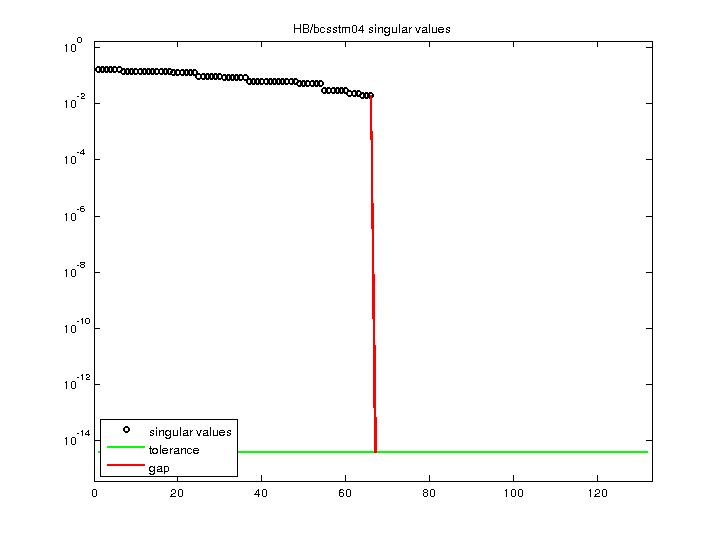Matrix: HB/bcsstm04

Description: SYMMETRIC MASS MATRIX, OIL RIG, NOT CONDENSED (SAME MODEL AS BCSSTX02)(undirected graph drawing)• Matrix group: HB
• download as a MATLAB mat-file, file size: 988 bytes. Use UFget(59) or UFget('HB/bcsstm04') in MATLAB.

 Matrix properties number of rows 132 number of columns 132 nonzeros 66 structural full rank? no structural rank 66 # of blocks from dmperm 68 # strongly connected comp. 132 explicit zero entries 66 nonzero pattern symmetry symmetric numeric value symmetry symmetric type real structure symmetric Cholesky candidate? no positive definite? no

 author J. Lewis editor I. Duff, R. Grimes, J. Lewis date 1982 kind structural problem 2D/3D problem? yes

 Ordering statistics: result nnz(chol(P*(A+A'+s*I)*P')) with AMD 132 Cholesky flop count 1.3e+02 nnz(L+U), no partial pivoting, with AMD 132 nnz(V) for QR, upper bound nnz(L) for LU, with COLAMD 132 nnz(R) for QR, upper bound nnz(U) for LU, with COLAMD 132

Note that all matrix statistics (except nonzero pattern symmetry) exclude the 66 explicit zero entries.

 SVD-based statistics: norm(A) 0.172829 min(svd(A)) 0 cond(A) Inf rank(A) 66 sprank(A)-rank(A) 0 null space dimension 66 full numerical rank? no singular value gap Inf

 singular values (MAT file): click here SVD method used: s = svd (full (A)) ; status: ok